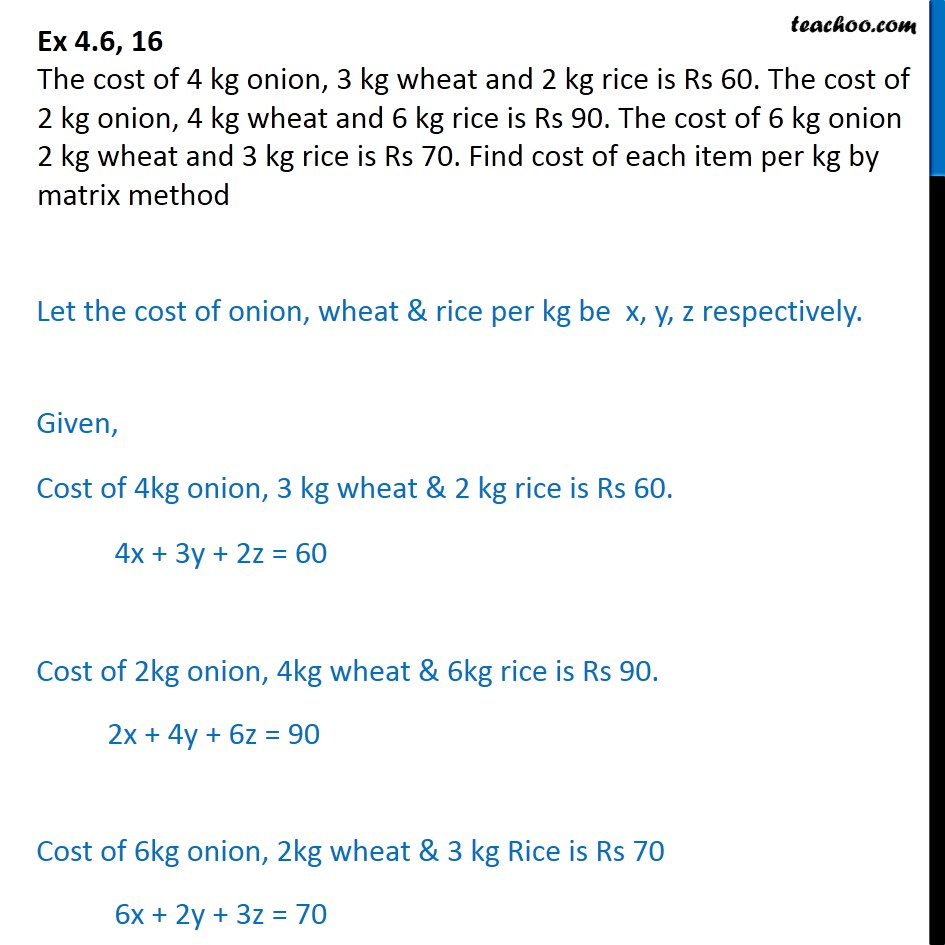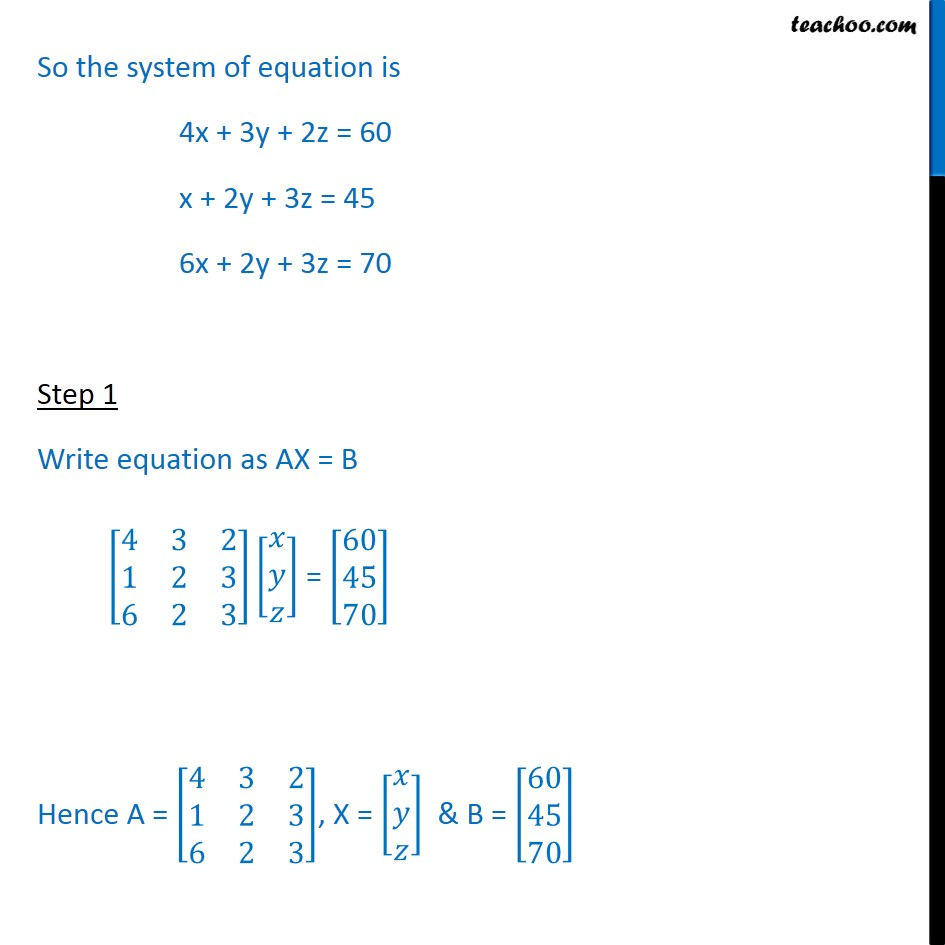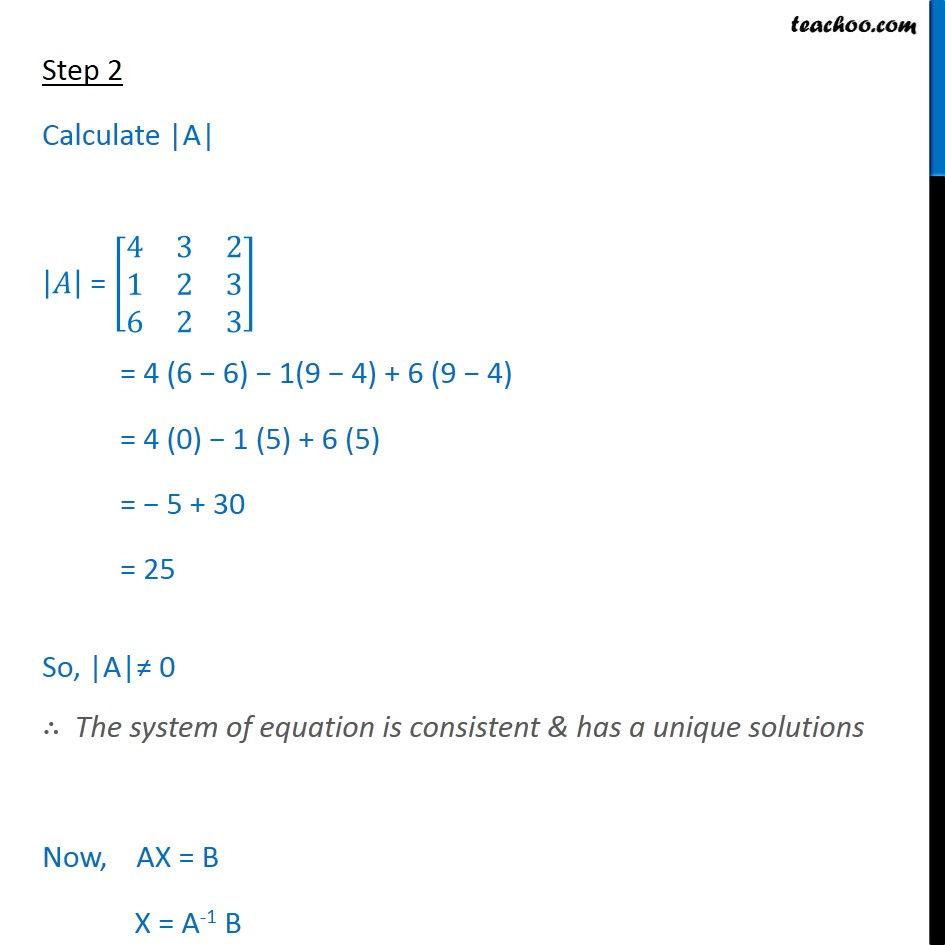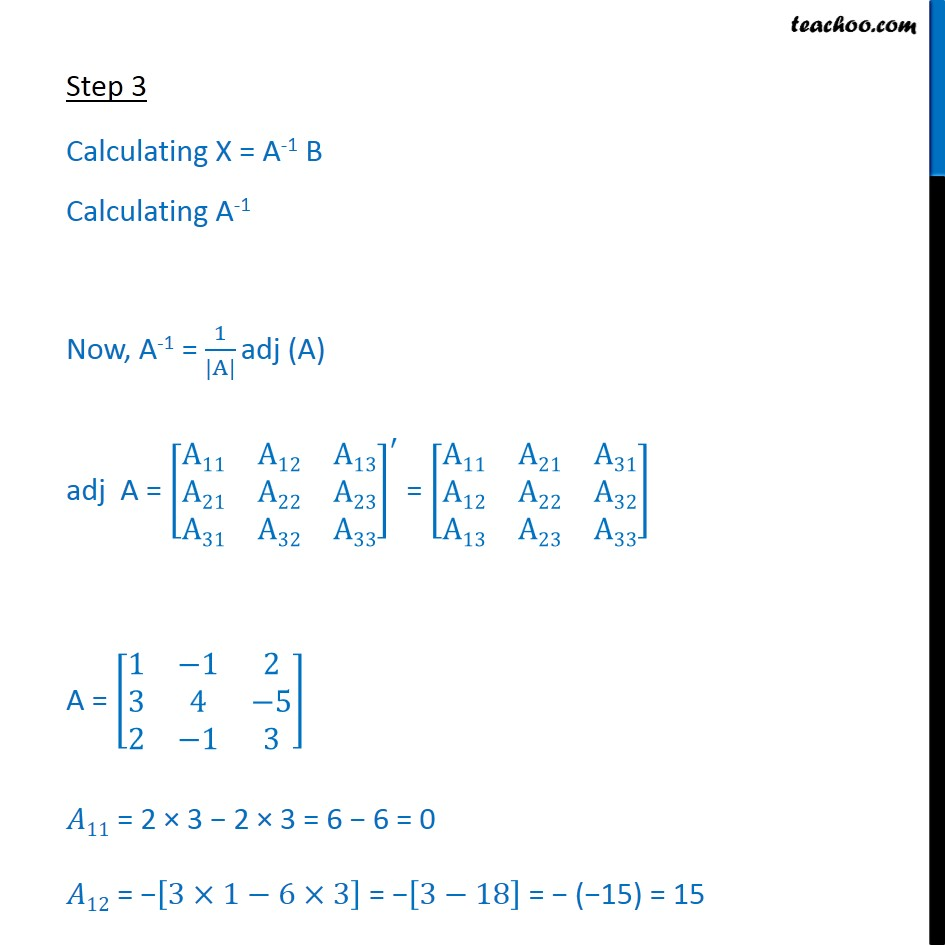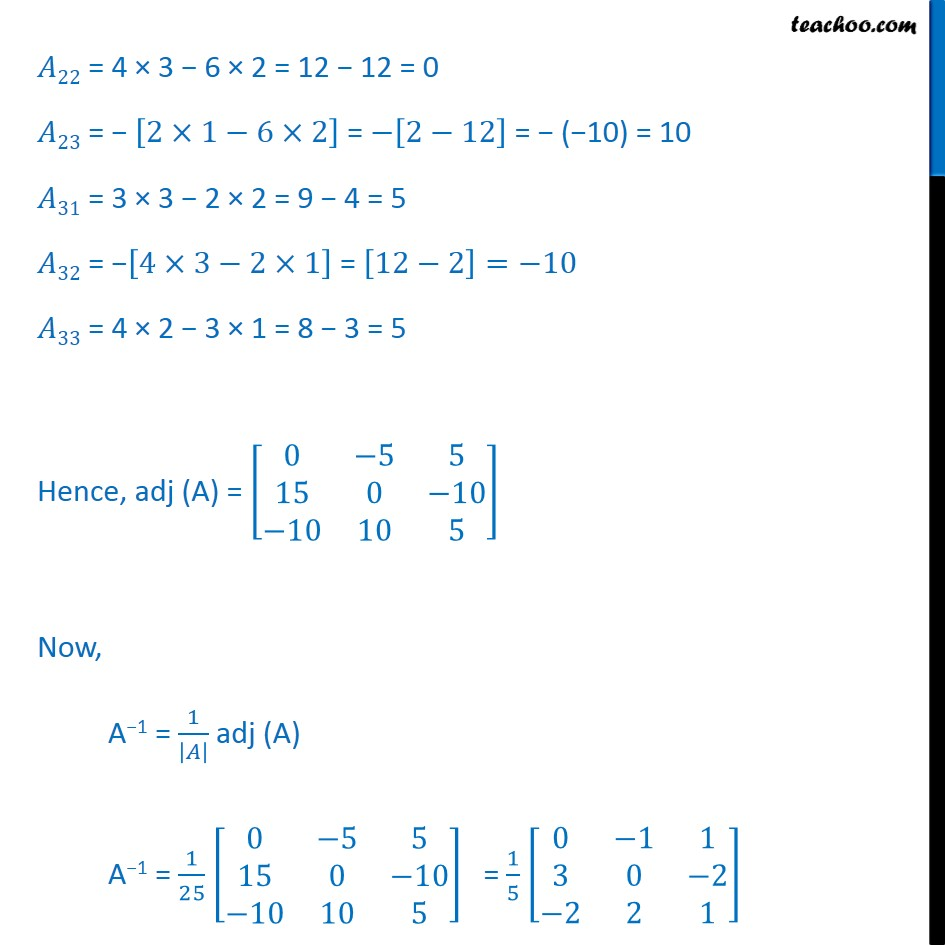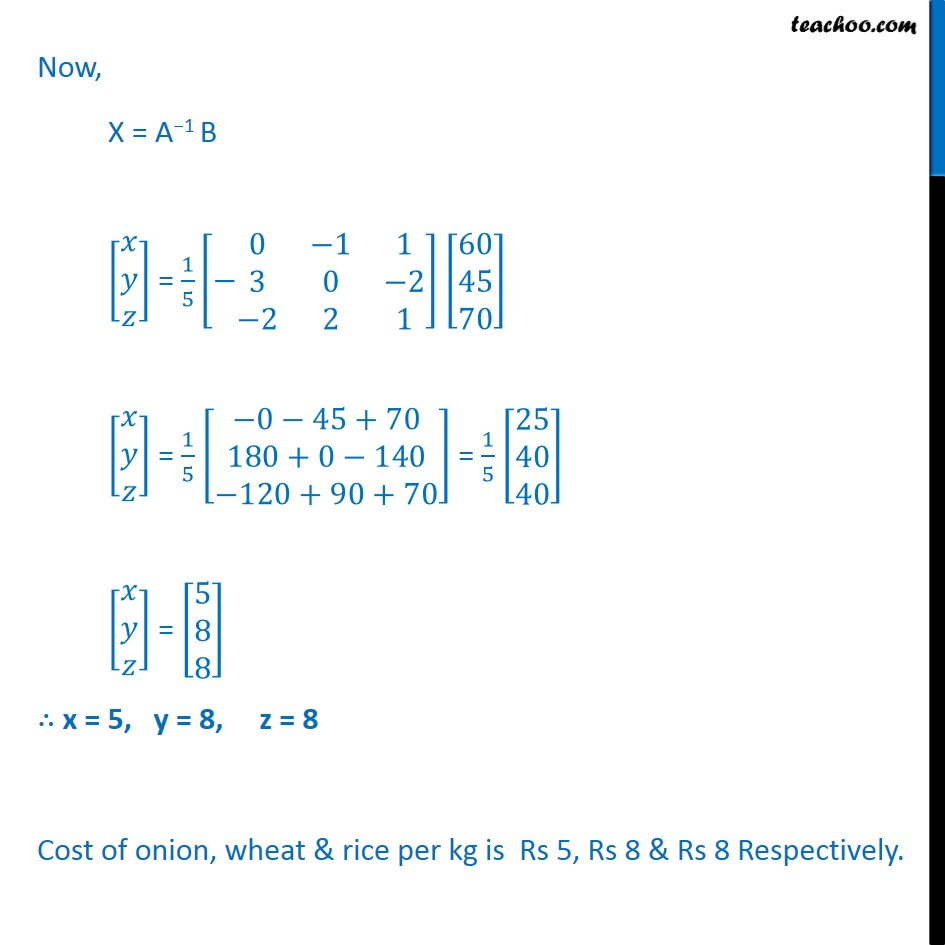1. Class 12
2. Important Question for exams Class 12
3. Chapter 4 Class 12 Determinants

Transcript

Ex 4.6, 16 The cost of 4 kg onion, 3 kg wheat and 2 kg rice is Rs 60. The cost of 2 kg onion, 4 kg wheat and 6 kg rice is Rs 90. The cost of 6 kg onion 2 kg wheat and 3 kg rice is Rs 70. Find cost of each item per kg by matrix method Let the cost of onion, wheat & rice per kg be x, y, z respectively. Given, Cost of 4kg onion, 3 kg wheat & 2 kg rice is Rs 60. 4x + 3y + 2z = 60 Cost of 2kg onion, 4kg wheat & 6kg rice is Rs 90. 2x + 4y + 6z = 90 Cost of 6kg onion, 2kg wheat & 3 kg Rice is Rs 70 6x + 2y + 3z = 70 So the system of equation is 4x + 3y + 2z = 60 x + 2y + 3z = 45 6x + 2y + 3z = 70 Step 1 Write equation as AX = B 4 3 2 1 2 3 6 2 3 = 60 45 70 Hence A = 4 3 2 1 2 3 6 2 3 , X = & B = 60 45 70 Step 2 Calculate |A| = 4 3 2 1 2 3 6 2 3 = 4 (6 6) 1(9 4) + 6 (9 4) = 4 (0) 1 (5) + 6 (5) = 5 + 30 = 25 So, |A| 0 The system of equation is consistent & has a unique solutions Now, AX = B X = A-1 B Step 3 Calculating X = A-1 B Calculating A-1 Now, A-1 = 1 |A| adj (A) adj A = A11 A12 A13 A21 A22 A23 A31 A32 A33 = A11 A21 A31 A12 A22 A32 A13 A23 A33 A = 1 1 2 3 4 5 2 1 3 11 = 2 3 2 3 = 6 6 = 0 12 = 3 1 6 3 = 3 18 = ( 15) = 15 22 = 4 3 6 2 = 12 12 = 0 23 = 2 1 6 2 = 2 12 = ( 10) = 10 31 = 3 3 2 2 = 9 4 = 5 32 = 4 3 2 1 = 12 2 = 10 33 = 4 2 3 1 = 8 3 = 5 Hence, adj (A) = 0 5 5 15 0 10 10 10 5 Now, A 1 = 1 adj (A) A 1 = 1 25 0 5 5 15 0 10 10 10 5 = 1 5 0 1 1 3 0 2 2 2 1 Now, X = A 1 B = 1 5 0 1 1 3 0 2 2 2 1 60 45 70 = 1 5 0 45+70 180+0 140 120+90+70 = 1 5 25 40 40 = 5 8 8 x = 5, y = 8, z = 8 Cost of onion, wheat & rice per kg is Rs 5, Rs 8 & Rs 8 Respectively.

Chapter 4 Class 12 Determinants

Class 12
Important Question for exams Class 12

About the AuthorDavneet Singh
Davneet Singh is a graduate from Indian Institute of Technology, Kanpur. He has been teaching from the past 9 years. He provides courses for Maths and Science at Teachoo.